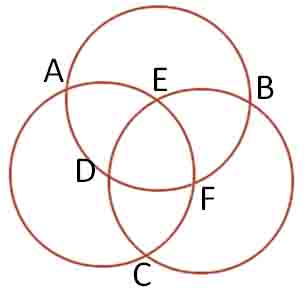BrainDen.com - Brain Teasers
• 0

# three circles

## Question

1. Easy Puzzle

Put numbers 1,2,3,5,6,7 to each point, so :

I.  The sum of points in every circle  are equal,  (A+E+F+C = B+E+D+C = A+D+F+B)

II.  A+B+C = D+E+F

There are 2 solutions.

2 .Harder Puzzle

Find the value of x, then put number 2,3,4,9,12,x to each point, so :

I.  The product of points in every circle  are equal,  (A*E*F*C = B*E*D*C = A*D*F*B)

II.  A*B*C = D*E*F

There are 2 solutions.## Recommended Posts

• 0
Spoiler

1. As the total of (1+2+3+5+6+7)/2 = 12, A+B+C = D+E+F = 12;
and the sum of each circle must be 16.
A B C D E F
1 5 6 3 2 7
1 6 5 2 3 6
2 3 7 5 1 6
2 7 3 1 5 6
3 2 7 6 1 5
3 7 2 1 6 5
5 1 6 7 2 3
5 6 1 2 7 3
6 1 5 7 3 2
6 5 1 3 7 2
7 2 3 6 5 1
7 3 2 5 6 1

2. As the prime factors of the known points are {2, 3, 2*2, 3*3, 2*2*3}, x must be 2*3*3 = 18.
As the square root of the product of 2*3*4*9*12*18 = 216, A*B*C = D*E*F = 216; the product of each circle is 1296 
A  B  C  D  E  F
2  9 12  4  3 18
2 12  9  3  4 18
3  4 18  9  2 12
3 18  4  2  9 12
4  3 18 12  2  9
4 18  3  2 12  9
9  2 12 18  3  4
9 12  2  3 18  4
12  2  9 18  4  3
12  9  2  4 18  3
18  3  4 12  9  2
18  4  3  9 12  2

##### Share on other sites

• 0

@DejMar

1st question
1 5 6 3 2 7 = 1 6 5 2 3 6 = 5 1 6 7 2 3 = 5 6 1 2 7 3 = 6 1 5 7 3 2 = 6 5 1 3 7 2   1st solution
2 3 7 5 1 6 = 2 7 3 1 5 6 = 3 2 7 6 1 5 = 3 7 2 1 6 5 = 7 2 3 6 5 1 = 7 3 2 5 6 1  2nd solution

2nd question
2  9 12  4  3 18 = 2 12  9  3  4 18 = 9  2 12 18  3  4 = 9 12  2  3 18  4 = 12  2  9 18  4  3 = 12  9  2  4 18  3 = 1st solution
3  4 18  9  2 12 = 3 18  4  2  9 12 = 4  3 18 12  2  9 = 4 18  3  2 12  9 = 18  3  4 12  9  2 = 18  4  3  9 12  2 = 2nd solution

Just Rotation and reflection of the circle.

##### Share on other sites

• 0

@jasen - Rotations and reflections may be considered the same for the visual layout, but not for the assignment of values to specific points. There are twelve different solutions. If one considers rotations and reflections to be pertinent, so one should one allow the eversion of this particular figure due to the placement of the points. In such case there is but one solution.

##### Share on other sites

• 0

Another "Easy" Puzzle

Find The value of x and y, then put numbers 2,4,8,32,x,y to each point, so :

I.  The product of points in every circle  are equal,  (A*E*F*C = B*E*D*C = A*D*F*B)
II.  A*B*C = D*E*F

There are 2 solutions (Reflection and rotation solutions are considered as 1 same solution)

• 0

### Exponent Laws:

xm * xn = xm+n

##### Share on other sites

• 0

Solution :

Spoiler

I call it Another "Easy" Puzzle, because it have a strong connection with my first question (Easy Puzzle).

use solution from my first answer :  A = 1, B = 5, C = 6, D = 3, E = 2, F = 7

then write it like this :A = 21 , B = 25 , C = 26 , D = 23 , E = 22 , F = 27

so (A,B,C,D,E,F) = (2,32,64,8,4,128)

This works by the Exponent Laws: xm * xn = xm+n

## Join the conversation

You can post now and register later. If you have an account, sign in now to post with your account.×   Pasted as rich text.   Paste as plain text instead

Only 75 emoji are allowed.

×   Your previous content has been restored.   Clear editor

×   You cannot paste images directly. Upload or insert images from URL.